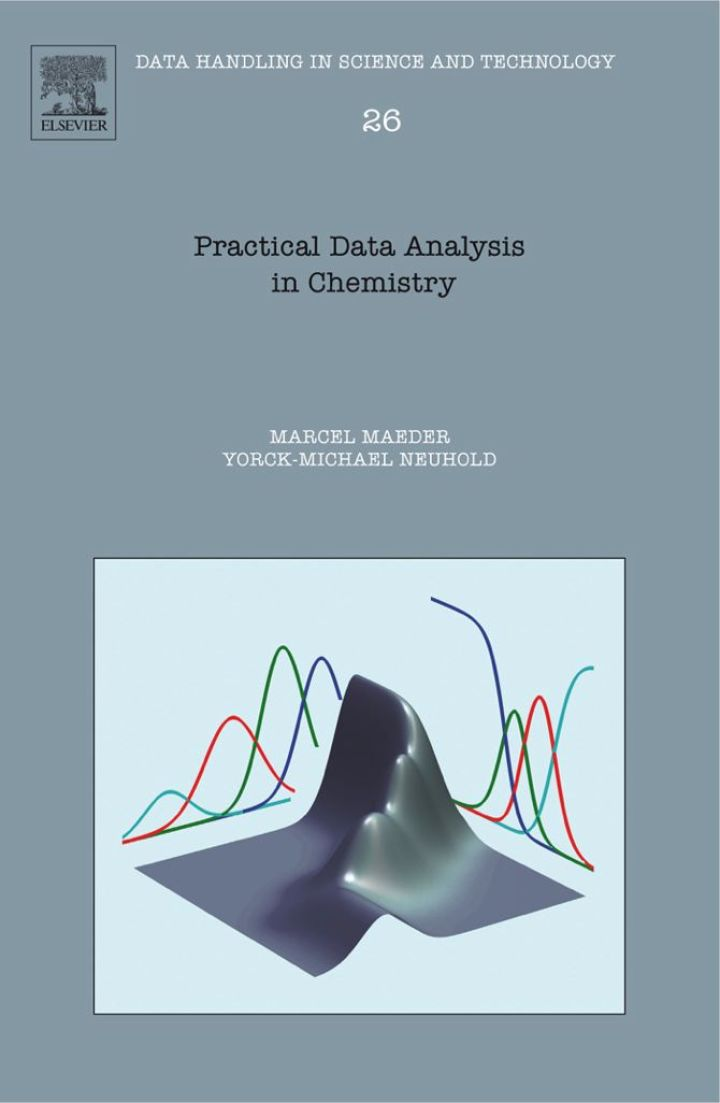# Practical Data Analysis in Chemistry

## Practical Data Analysis in Chemistry

By Maeder, Marcel; Neuhold, Yorck-Michael

### Rent or Buy eTextbook

Expires on Apr 14th, 2022
\$185

Publisher List Price: \$189.99
Savings: \$4.99

The majority of modern instruments are computerised and provide incredible amounts of data. Methods that take advantage of the flood of data are now available; importantly they do not emulate 'graph paper analyses' on the computer. Modern computational methods are able to give us insights into data, but analysis or data fitting in chemistry requires the quantitative understanding of chemical processes. The results of this analysis allows the modelling and prediction of processes under new conditions, therefore saving on extensive experimentation. Practical Data Analysis in Chemistry exemplifies every aspect of theory applicable to data analysis using a short program in a Matlab or Excel spreadsheet, enabling the reader to study the programs, play with them and observe what happens. Suitable data are generated for each example in short routines, this ensuring a clear understanding of the data structure. Chapter 2 includes a brief introduction to matrix algebra and its implementation in Matlab and Excel while Chapter 3 covers the theory required for the modelling of chemical processes. This is followed by an introduction to linear and non-linear least-squares fitting, each demonstrated with typical applications. Finally Chapter 5 comprises a collection of several methods for model-free data analyses.

* Includes a solid introduction to the simulation of equilibrium processes and the simulation of complex kinetic processes.
* Provides examples of routines that are easily adapted to the processes investigated by the reader
* 'Model-based' analysis (linear and non-linear regression) and 'model-free' analysis are covered

Subject: Mathematics & Statistics -> Mathematics -> Mathematics GeneralPublisher: Elsevier S & T 08/2007
Imprint: Elsevier Science
Language: English
Length: 340 pages

ISBN 10: 0444530541
ISBN 13: 9780444530547
Print ISBN: 9780444530547

Live Chats